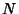## Sublime Number

Letanddenote the number and sum of the divisors of, respectively (i.e., the zeroth- and first-order Divisor Functions). A numberis called sublime ifandare both Perfect Numbers. The only two known sublime numbers are 12 andIt is not known if any Odd sublime number exists.